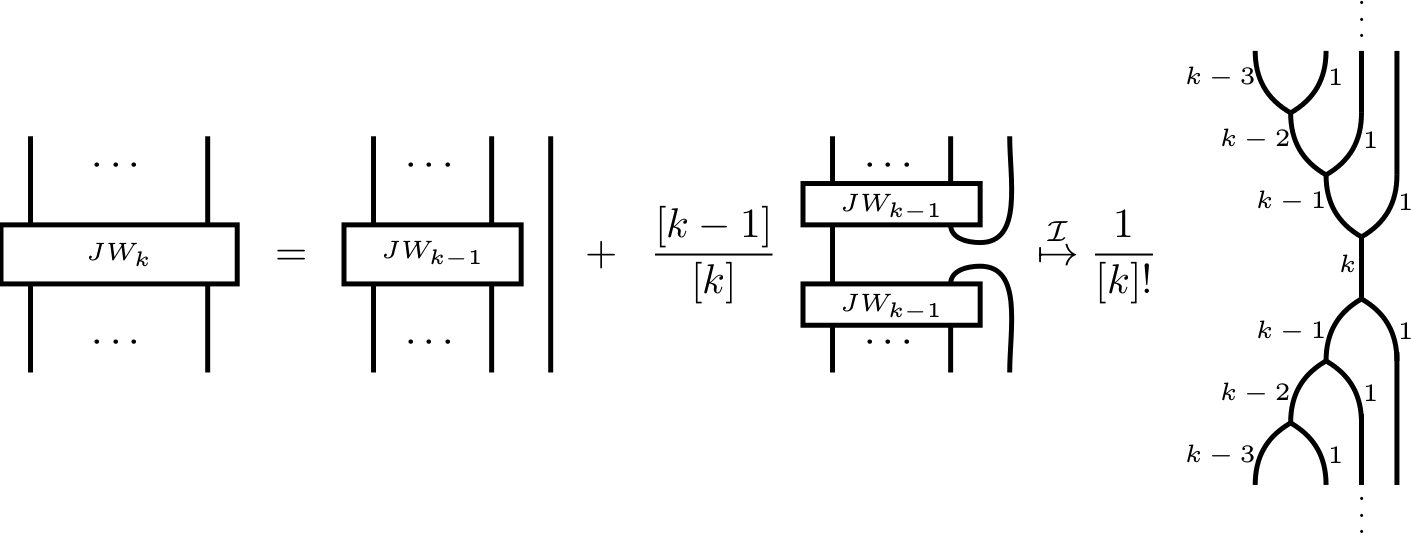## Abstract

We define and study the category of symmetric $$\mathfrak{sl}_2$$-webs. This category is a combinatorial description of the category of all finite dimensional quantum $$\mathfrak{sl}_2$$-modules. Explicitly, we show that (the additive closure of) the symmetric $$\mathfrak{sl}_2$$-spider is (braided monoidally) equivalent to the latter. Our main tool is a quantum version of symmetric Howe duality. As a corollary of our construction, we provide new insight into Jones-Wenzl projectors and the colored Jones polynomials.

## A few extra words

A classical result of Rumer, Teller and Weyl, modernly interpreted, states that the so-called Temperley-Lieb category $$\mathcal{TL}$$ describes the full subcategory of quantum $$\mathfrak{sl}_2$$-modules generated by tensor products of the 2-dimensional vector representation V of quantum $$\mathfrak{sl}_2$$.
By Karoubi completion, we get the whole category of finite dimensional $$\mathfrak{sl}_2$$-modules. Thus, it is a striking question if one can give a diagrammatic description of $$\mathbf{KAR}(\mathcal{TL})$$ as well.
We provide a new diagrammatic description of the entire category of finite dimensional quantum $$\mathfrak{sl}_2$$-modules.
To this end, we introduced our new description of the representation theory of quantum $$\mathfrak{sl}_2$$, the category of symmetric $$\mathfrak{sl}_2$$-webs akin to the category of “usual” $$\mathfrak{sl}_n$$-webs studied by many people (recall that these “usual” $$\mathfrak{sl}_n$$-webs give a diagrammatic presentation of the full subcategory of all finite dimensional $$\mathfrak{sl}_n$$-modules whose objects are finite tensor products of the fundamental $$\mathfrak{sl}_n$$-representations.
Our main tool is the usage of symmetric q-Howe duality.
In particular, the Jones-Wenzl projectors are included in our picture, but without any recursive formula. Namely, they are directly given as below.
Another corollary of our construction is a “MOY-calculus” for colored Jones polynomials.